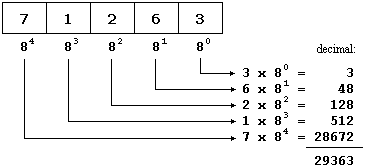Problems
302 - Octal to Decimal

Octal to Decimal

Time Limit: 1 sec

The Problem

The octal numeral system, or oct for short, is the base-8 number system, and uses the digits 0 to 7.

Write a simple program which read a 5 digit octal number X and convert the octal number to decimal then print the decimal number.

Example:

if X = 71263

Calculation is very simple

7 X 84 +  1 X 83 + 2 X 82 + 6 X 81 + 3 X 20

= 7 X 4096 + 1 X 512 + 2 X 64 + 6 X 8 + 3 X 1

= 28672 + 512 + 128 + 48 + 3

= 29363The Input

The input file contains only one 5 digit octal number.

The Output

Output will show the one integer number wich is the converted decimal value from the given octal number in a separate line.

Sample Input

71263

Sample Output

29363

Problem Setter: Shahin ShamS